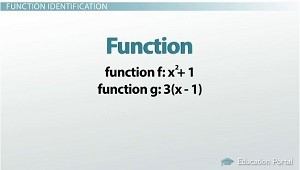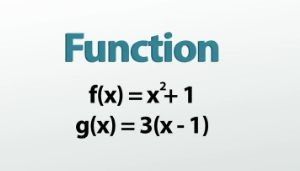# Functions: Identification, Notation

#### Video Lesson on Functions: Identification, Notation

This lesson video Not available at this time available video coming soon

### Functions: Identification, Notation & Practice Problems

A function is simply a rule that takes one number and turns it into another. But some special conditions must apply for it to be a true mathematical function. Learn about those conditions and how we write functions here!

### FunctionsF and g are most commonly used to name functions.

The word function gets thrown around a lot in algebra class, but it can often catch you off guard. Wait, what? Function? Where did that come from? Well, the reason math teachers use it so much and often don't even realize they're doing it is that the word function is really just a fancy word for 'equation' or 'rule.' A function is something you plug one thing into and get another thing out.

There are actually functions all around you! If you take tests, there is a function that tells you if you get this score on your test, you will get this grade. If you go grocery shopping, there is a function that tells you if you buy this many bottles of water, you need to pay this much money. If you pay for cable TV, there is a function that tells you if you want this many channels, you need to pay this much money.

### Function Identification

While a function doesn't necessarily have to use numbers, this is a math class, so the rest of the examples we'll look at will involve numbers - like these for example: x^2 + 1 and 3(x - 1). So we've got two functions (or rules or equations), but it's nice having names for these functions so we can be clear about which one we're talking about. The two most common names for mathematical functions are f and g. Let's name this one f (x^2 + 1) and this other one g (3(x - 1)).

What do we do with functions? We plug numbers into them and see what pops out! When we plug, say, 5, into the f function, we'd get 26 out. Or if we plugged -2 into the g function, we'd get -9 out.

As you can see, we've got inputs and outputs. In most areas of math, we use x for the inputs and y for the outputs, but functions are a little bit different. If we just said, we plugged in x = 5 and got out y = 26, it's not obvious which function we used to get that. Now, it probably wouldn't be too hard to figure out, but it would be nice if it was obvious. Also, just looking at the output, y = 26, doesn't give us any clues about what we plugged in to begin with to get that number.

So, mathematicians came up with a different way of writing the outputs to make these things more informative. Now, this does make it more confusing at first, but once you get it, it's actually pretty helpful.

Okay, so instead of calling the outputs y, the outputs from the f function will be called f(x), and the outputs from the g function will be called g(x). Notice that I'm saying f of x or g of x. I'm not saying f times x. It can be easy to see the parentheses and think multiply, but if it's an f or a g, resist the urge!F(x) is basically just a y output.

Now, when we plug 5 into the f function, instead of saying y = 26, we say f(5) = 26. This tells us that we used the f function and 5 was our input - much more informative! By this same logic, g(-2) = -9. Also, now instead of having to say the f function is x^2 + 1 and the g function is 3(x - 1), we can simply write f(x) = x^2 + 1 and g(x) = 3(x - 1). Again, these are basically the same as y =, so if you see an f(x) and get freaked out, don't worry - it's basically just a y.

This way of writing f(x) or g(x) instead of y is called function notation and is going to be used throughout math and science classes from now on.

Before we finish, I have to clarify one thing I said earlier. When I said that a function was just another way of saying equation or rule, I wasn't being entirely honest. The reason is that functions are a special breed of equation. Not every equation gets to be a function - there is a test you have to pass first. In order to illustrate what that test is, I'm going to use an analogy.

### Enjoy Slurp

Let's say we've got a soda machine. It could be called a function, right? I mean, you push the button telling it what kind of soda you want - the input - and then it spits one out - the output. Alright, well, for lack of a better word, let's say this is a Slurp machine. It has three buttons on top that will all give you regular Slurp, two buttons for Diet Slurp, one for Dr. Slurp, one for Extreme Slurp and finally one for Fruity Slurp. This is pretty normal to see. There are three for regular Slurp because that's the most popular one, so there needs to be a bunch of it. Diet Slurp is pretty popular, so it gets two, while the rest only have one because they don't sell as well.

Now let's say we're going to use this machine. You and I walk up to it and both decide to get Dr. Slurp. You push the Dr. Slurp button, and your Dr. Slurp pops out - cool. Now I do the same thing - push the same Dr. Slurp button - but then Extreme Slurp comes out! What? That's not what I asked for! You pushed Dr. Slurp, and it gave you what you wanted, but when I pushed the exact same button, I got Extreme Slurp! This machine is broken. I want my money back!

Okay. I'll calm back down and re-focus. The reason I tell this story is that functions are just like the Slurp machine was. They're allowed to have multiple buttons that give you the same thing, but if one button sometimes gives you this and sometimes gives you that, it's broken and not a function at all.

Saying this in math sounds like this: multiple inputs can have the same output, but one input cannot lead to multiple outputs. Each input must have a unique output. Let's look at a few example problems and decide whether or not they are functions.

### Function and Relation Examples

The most basic type of function we can have is just a pairing of input values with output values, like this: (2,3) (5,6) (7,8). This tells us that when 2 is plugged in, 3 is the output; when 5 is plugged in, 6 is the output; and so on. We can agree that this is a function because each input always gives you the same output. 2 always gives you 3, 5 always gives you 6, and 7 always gives you 8.

This one (2,1) (3,1) (4,1) (5,1) (6,2) is also okay because each input always gives you the same thing. Remember that it is okay to have multiple buttons that give you the same thing, like you see here with 2, 3, 4 and 5 all giving you 1.

But here (1,2) (1,3) (1,4) (2,5) we run into a problem because the input 1 has multiple outputs. Sometimes 1 will give you 2, but sometimes it'll give you 3 and sometimes even 4. Since it is not a function, it is simply called a 'relation.'

### Vertical Line TestingThis graph is not a function because there are three spots on the line that go with the x-value.

We can also tell whether or not something is a function by looking at its graph. Here, I know that this is a function because for any x-value I pick, there is only one spot on the line that goes with that value. But for this graph now, right away I can tell it is not a function because for any x-value right in the middle here, there are actually three different spots on the line that go with it. Even though these parts of the graph are okay, the fact that this part here in the middle exists ruins it all, and it's therefore not a function.

What we are doing here is called the vertical line test. It's basically just a trick you can use to see whether a graph is a function or not. By dragging an imaginary vertical line over the graph and checking to see if there is ever a point where your line touches the graph in two places, you can tell if it's a function. If the line ever, even just once, touches the graph in two or more spots, it is not a function. If this isn't the c

### Next Topics